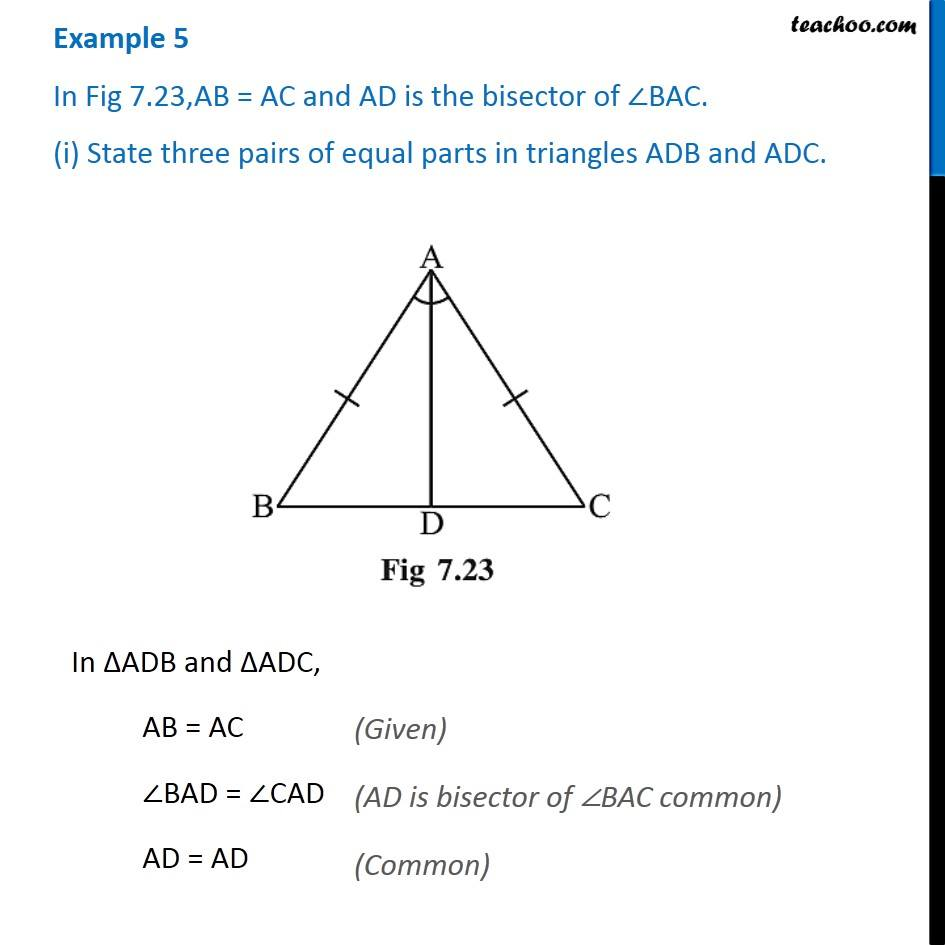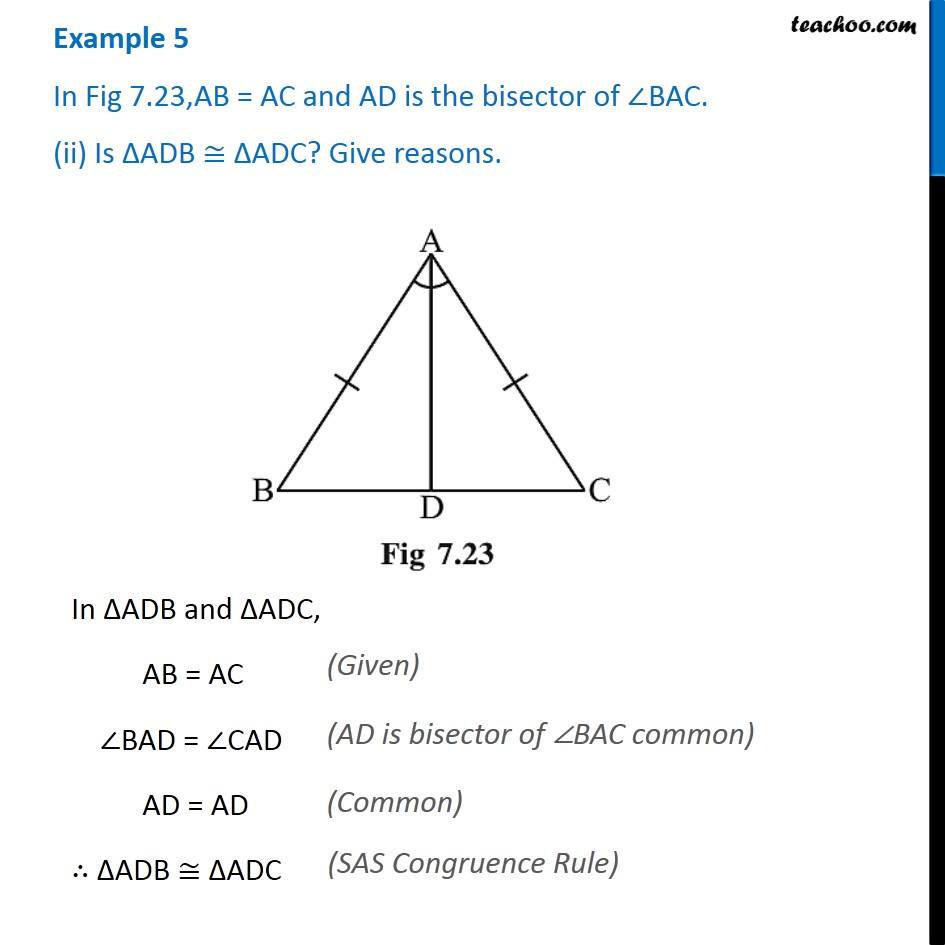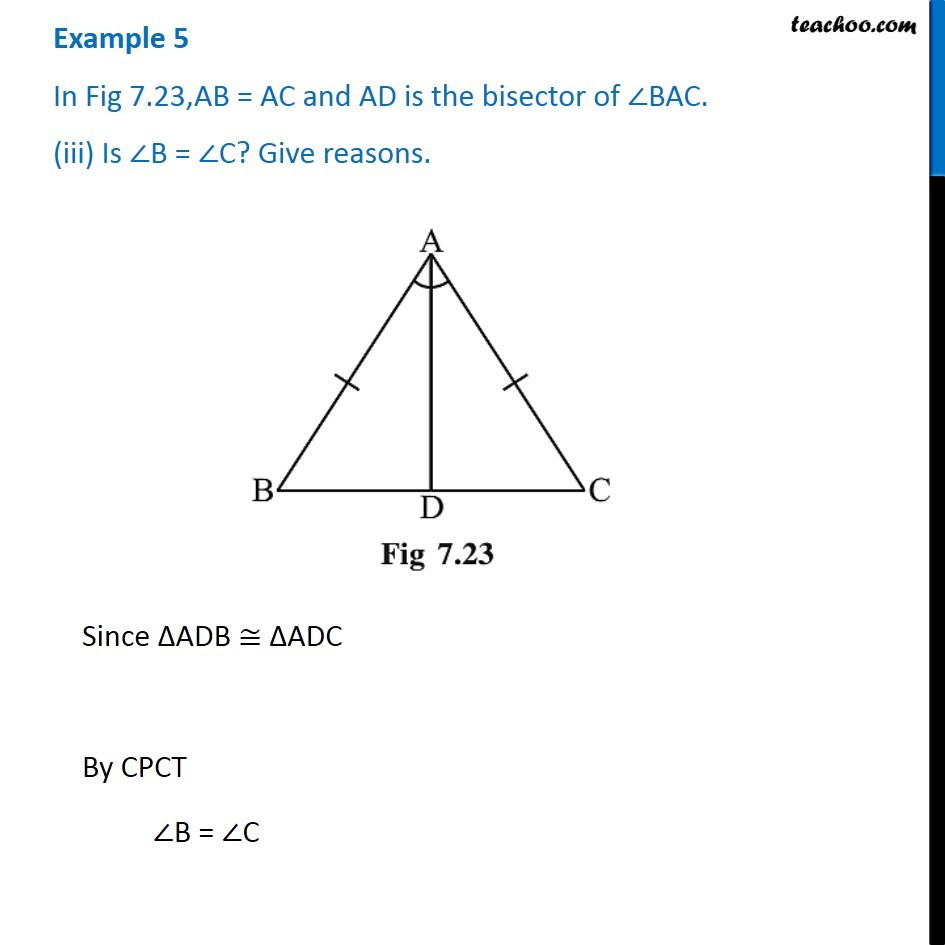1. Chapter 7 Class 7 Congruence of Triangles
2. Serial order wise
3. Examples

Transcript

Example 5 In Fig 7.23,AB = AC and AD is the bisector of ∠BAC. (i) State three pairs of equal parts in triangles ADB and ADC. In ∆ADB and ∆ADC, AB = AC ∠BAD = ∠CAD AD = AD Example 5 In Fig 7.23,AB = AC and AD is the bisector of ∠BAC. (ii) Is ∆ADB ≅ ∆ADC? Give reasons.In ∆ADB and ∆ADC, AB = AC ∠BAD = ∠CAD AD = AD ∴ ∆ADB ≅ ∆ADC Example 5 In Fig 7.23,AB = AC and AD is the bisector of ∠BAC. (iii) Is ∠B = ∠C? Give reasons.Since ∆ADB ≅ ∆ADC By CPCT ∠B = ∠C

Examples

About the AuthorDavneet Singh
Davneet Singh is a graduate from Indian Institute of Technology, Kanpur. He has been teaching from the past 9 years. He provides courses for Maths and Science at Teachoo.